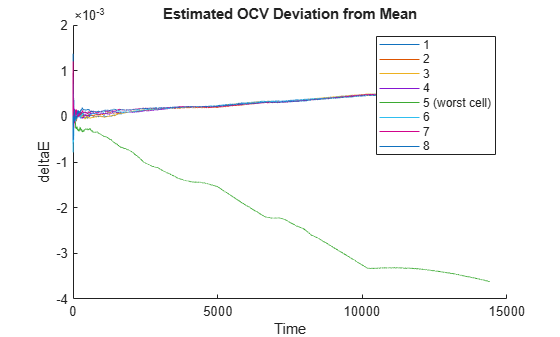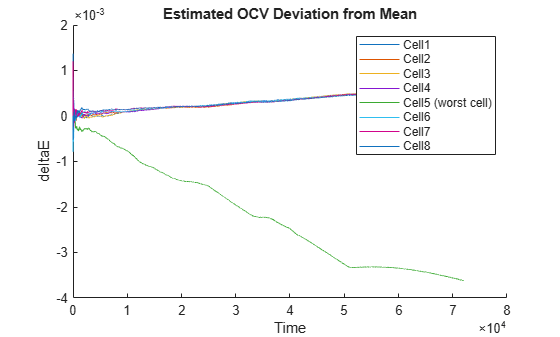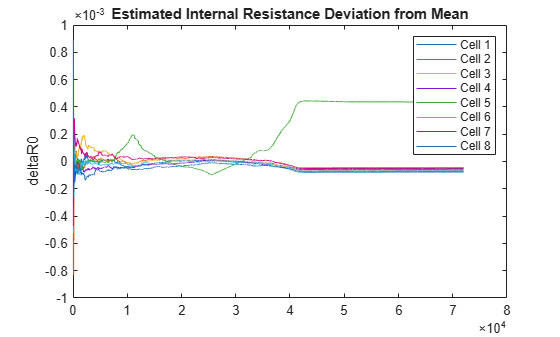# meanDifferenceModel

Identify most degraded cell in serially connected lithium-ion battery pack

## Syntax

``meanDifferenceModel(data)``
``meanDifferenceModel(data,Name=Value)``
``[worstcell,deltae,deltar0] = meanDifferenceModel(___)``

## Description

example

````meanDifferenceModel(data)` analyzes the consistency of the open-circuit voltage (OCV) for each cell in a serially connected lithium-ion battery pack and plots the results. The software combines the recursive least square (RLS) algorithm with the mean-difference model (MDM) to estimate the deviation of the OCV of each cell from the mean OCV of the pack . The larger the deviation is in the negative direction, the more likely it is that the battery pack has a fault condition, such as an internal short circuit. The plot legend identifies the cell with the highest deviation, and therefore, the worst cell in the pack.The algorithm imposes no restrictions on battery activity during the collection of `data`. The battery can be in any operating sequence, including charging, discharging, and standby phases.```

example

````meanDifferenceModel(data,Name=Value)` incorporates additional options specified by one or more name-value arguments. For example, set the `CurrentVariable` argument to `10` to specify that the column with index `10` contains the pack current values.```

example

````[worstcell,deltae,deltar0] = meanDifferenceModel(___)` returns the index of the worst cell `worstcell`, the deviation `deltae` of the cell voltages from the mean OCV, and the deviation `deltar0` of the internal resistances from the mean R0 value. You can use this syntax with any of the previous input-argument combinations.```

## Examples

collapse all

Load the data, which represents an operating profile for an eight-cell battery pack in which one cell is experiencing an internal short circuit. The profile includes standby, driving, charging, and balancing phases. The data consists of 10 columns that contain the sample time (column 1), battery voltages (columns 2–9), and pack current (column 10).

`load internalShortCircuit.mat internalShortCircuit`

Plot the battery voltages and the pack current together.

```plot(internalShortCircuit(:,2:9)) hold on plot(internalShortCircuit(:,10)) legend('Voltages (V)','Current (A)') title('internalShortCircuit Datasets - Voltages and Pack Current') ylabel('Voltage (V) and Current (A) Values') hold off```The voltages appear to track closely, but at this scale it is hard to differentiate them.

Zoom into the region after t = 4000 to show the individual voltages more clearly.

```xlim([4000 4010]) ylim([3.4 3.6]) title('Voltage Separation') ylabel('Voltages (V)')```The voltages track closely together. There is no cell that is obviously degrading relative to the others.

Identify the worst cell using `meanDifferenceModel`.

`meanDifferenceModel(internalShortCircuit(:,2:end))`The mean difference model clearly differentiates cell 5.

Load the data, which is contained in a table, and show the first two rows.

```load internalShortCircuitTbl.mat internalShortCircuitTbl head(internalShortCircuitTbl,2)```
``` Time Cell1 Cell2 Cell3 Cell4 Cell5 Cell6 Cell7 Cell8 Current ____ ______ ______ _____ ______ ______ ______ ______ _____ _______ 0 0 0 0 0 0 0 0 0 0 5 4.0037 4.0018 4.002 4.0012 4.0029 4.0012 4.0042 4.002 0 ```
`data = internalShortCircuitTbl;`

Use `meanDifferenceModel` to identify the worst cell, specifying the time and current variables. Use `Time` to specify the time variable. Use `CurrentVariable` to specify the current variable.

`meanDifferenceModel(data(:,2:end),Time=data.Time,CurrentVariable="Current")`The plot identifies the worst cell.

Load the data, which is contained in a table.

```load internalShortCircuitTbl.mat internalShortCircuitTbl data = internalShortCircuitTbl;```

Run `meanDifferenceModel`, using output arguments to store the analysis results.

`[worstcell,deltae,deltar0] = meanDifferenceModel(data(:,2:end),Time=data.Time,CurrentVariable="Current");`

Identify the worst cell.

`iwc = worstcell`
```iwc = 5 ```

Plot the estimated internal resistance deviations.

```time = data.Time; plot(data.Time,deltar0.Variables) title('Estimated Internal Resistance Deviation from Mean') legend("Cell 1","Cell 2","Cell 3","Cell 4","Cell 5","Cell 6","Cell 7","Cell 8") ylabel('deltaR0')```Cell 5, which the function identifies as the worst cell, has the largest internal resistance deviation.

## Input Arguments

collapse all

Battery cell voltage and pack current data, specified as a `table`, a `timetable`, or a matrix that contains ns rows, where ns is the number of samples, and nc+1 columns or variables, where nc is the number of battery cells. By default, the software interprets the last column as the pack current. You can specify a different column for the pack current by using the `'CurrentVariable'` name-value argument.

If your measurement data also includes an explicit column for time, you must exclude that column from the `data` argument when you call `meanDifferenceModel`. However, you can access the time data for plotting by using the `'Time'` name-value argument.

Example: `meanDifferenceModel(data(:,2:end),Time=data(:,1))` analyzes the OCV deviations and pack current using the voltage and current data in the matrix or table `data`, and plots the results against the time data in the first column.

### Name-Value Arguments

Specify optional pairs of arguments as `Name1=Value1,...,NameN=ValueN`, where `Name` is the argument name and `Value` is the corresponding value. Name-value arguments must appear after other arguments, but the order of the pairs does not matter.

Example: `meanValueModel(data,CurrentVariable="Current")` identifies the table or timetable variable with the name `"Current"` as the pack current variable.

Time data to use for plotting, specified as numeric vector, a `duration` vector, or a `datetime` vector of length ns, where ns is the number of samples in `data`. `'Time'` can be a column in the dataset used for `data`, or a separate vector.

If `data` is a `timetable`, the time information in `data` takes precedence and the software ignores the `'Time'` specification.

If `data` is a table or a matrix and `'Time'` is not specified, then the function plots the results against the index vector [1:ns].

Example: `meanDifferenceModel(data,Time=tv)` specifies the time vector `tv`, which has the same number of rows as `data` but is separate from `data`.

Pack-current column identifier that provides the variable name or column index of the pack-current data, specified as a string, a character vector, or an integer.

• When `data` is a timetable or table, `'CurrentVariable'` can be a string, a character vector, or an integer.

• When `data` is a matrix, `'CurrentVariable'` must be an integer.

Example: `meanValueModel(data,CurrentVariable=10)` identifies the column with the index of `10` as the pack current data.

Forgetting factor for RLS (recursive least squares) estimation, specified as a numeric scalar in the range (0,1]. The value of `'ForgettingFactor'` determines how significant the past measurements are for parameter estimation. A value of `1` corresponds to "no forgetting", that is, maintaining the values of all past measurements. For more information, see the ` ForgettingFactor` argument description in `recursiveLS`

Example: `meanValueModel(data,ForgettingFactor=0.995)` sets the forgetting factor to 0.995.

## Output Arguments

collapse all

Index of the worst cell, returned as an integer that corresponds to the column of `data` that contains the voltage of the cell for which the OCV deviation from the mean OCV is the greatest in the negative direction.

Estimated OCV deviations from the mean OCV, returned as a table, timetable, or matrix. The data type of `deltae` is consistent with the data type of `data`.

Estimated internal resistance deviations from the mean internal resistance R0, returned as a table, timetable, or matrix. The data type of `deltar0` is consistent with the data type of `data`.

 Ouyang, Minggao, Mingxuan Zhang, Xuning Feng, Languang Lu, Jianqiu Li, Xiangming He, and Yuejiu Zheng. “Internal Short Circuit Detection for Battery Pack Using Equivalent Parameter and Consistency Method.” Journal of Power Sources 294 (October 2015): 272–83. https://doi.org/10.1016/j.jpowsour.2015.06.087.